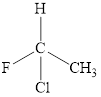Chapter 23.2, Problem 23.1CYU

Chapter
Section
Textbook Problem

(a) Draw the nine isomers having the formula C7H16. (Hint: There is one structure with a seven-carbon chain, two structures with six-carbon chains [one is illustrated in the margin above], five structures with a five-carbon chain, and one structure with a four-carbon chain.)(b) Identify the isomers of C7H16 that are chiral.

(a)

Interpretation Introduction

Interpretation: (a)The structural isomers of the given alkane C7H16 has to be drawn. (b)The chiral structures among them are to be identified.

Concept introduction: Structural Isomers are the structure of a molecule with same molecular formula but have different arrangements of bonds and atoms. For a single molecular formula of alkanes higher than propane different arrangements of atoms can give a different structure.

A chiral carbon is the carbon atom which is substituted to four different groups. Due to presence of chiral carbon a molecule become chiral and it gives optical isomers also that is R and S isomers. For example,All the substituents are different hence is is a chiral carbon and so molecule.

Explanation

The simplest structure of given alkane is with linear carbon chain of seven carbons, two structure can be formed  from six carbon chain, five structures with five carbon chain and  four carbon chain also give one structure.

Given:

The molecular formula of given alkane is C7H16. The all structural isomers for this can be drawn as follows.

(1) Seven carbons parent chain with no side chain.

(2) Six carbons parent chain with one methyl side chain two structures can be formed.

(b)

Interpretation Introduction

Interpretation: The chiral structures among the structural isomers of the given alkane C7H16 are to be identified.

Concept introduction: Structural Isomers are the structure of a molecule with same molecular formula but have different arrangements of bonds and atoms. For a single molecular formula of alkanes higher than propane different arrangements of atoms can give a different structure.

A chiral carbon is the carbon atom which is substituted to four different groups. Due to presence of chiral carbon a molecule become chiral and it gives optical isomers also that is R and S isomers. For example,All the substituents are different hence is is a chiral carbon and so molecule.

Still sussing out bartleby?

Check out a sample textbook solution.

See a sample solution

The Solution to Your Study Problems

Bartleby provides explanations to thousands of textbook problems written by our experts, many with advanced degrees!

Get Started